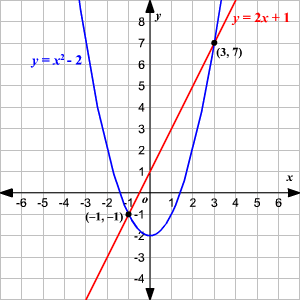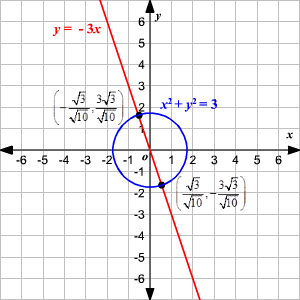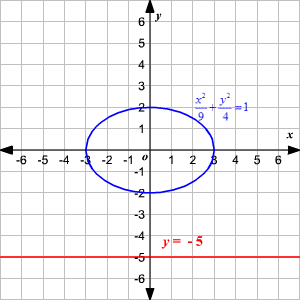You have probably solved systems of linear equations. But what about a system of two equations where one equation is linear, and the other is quadratic?

We can use a version of the substitution method to solve systems of this type.

Remember that the slope-intercept form of the equation for a line is $y=mx+b$, and the standard form of the equation for a parabola with a vertical axis of symmetry is $y=a{x}^{2}+bx+c,\text{\hspace{0.17em}}\text{\hspace{0.17em}}a\ne 0$.

To avoid confusion with the variables, let us write the linear equation as $y=mx+d$ where $m$ is the slope and $d$ is the $y$-intercept of the line.

Substitute the expression for $y$ from the linear equation, in the quadratic equation. That is, substitute $mx+d$  for $y$ in $y=a{x}^{2}+bx+c$ .

$mx+d=a{x}^{2}+bx+c$

Now, rewrite the new quadratic equation in standard form.

Subtract $mx+d$  from both sides.

$\begin{array}{l}\left(mx+d\right)-\left(mx+d\right)=\left(a{x}^{2}+bx+c\right)-\left(mx+d\right)\\ 0=a{x}^{2}+\left(b-m\right)x+\left(c-d\right)\end{array}$

Now we have a quadratic equation in one variable, the solution of which can be found using the quadratic formula.

The solutions to the equation $a{x}^{2}+\left(b-m\right)x+\left(c-d\right)=0$   will give the $x$-coordinates of the points of intersection of the graphs of the line and the parabola. The corresponding $y$-coordinates can be found using the linear equation.

Another way of solving the system is to graph the two functions on the same coordinate plane and identify the points of intersection.

Example 1:

Find the points of intersection between the line $y=2x+1$  and the parabola $y={x}^{2}-2$.

Substitute $2x+1$  for $y$ in $y={x}^{2}-2$.

$2x+1={x}^{2}-2$

Write the quadratic equation in standard form.

$\begin{array}{l}2x+1-2x-1={x}^{2}-2-2x-1\\ 0={x}^{2}-2x-3\end{array}$

Use the quadratic formula to find the roots of the quadratic equation.

Here, $a=1,\text{\hspace{0.17em}}\text{\hspace{0.17em}}b=-2,\text{\hspace{0.17em}}$ and $c=-3$.

$\begin{array}{l}x=\frac{-\left(-2\right)\text{\hspace{0.17em}}±\text{\hspace{0.17em}}\sqrt{{\left(-2\right)}^{2}\text{\hspace{0.17em}}-\text{\hspace{0.17em}}4\left(1\right)\left(-3\right)}}{2\left(1\right)}\\ =\frac{2\text{\hspace{0.17em}}±\text{\hspace{0.17em}}\sqrt{4\text{\hspace{0.17em}}+\text{\hspace{0.17em}}12}}{2}\\ =\frac{2\text{\hspace{0.17em}}±\text{\hspace{0.17em}}4}{2}\\ =3,\text{\hspace{0.17em}}\text{\hspace{0.17em}}-1\end{array}$

Substitute the $x$-values in the linear equation to find the corresponding $y$-values.

$\begin{array}{l}x=3⇒y=2\left(3\right)+1\\ \text{\hspace{0.17em}}\text{\hspace{0.17em}}\text{\hspace{0.17em}}\text{\hspace{0.17em}}\text{\hspace{0.17em}}\text{\hspace{0.17em}}\text{\hspace{0.17em}}\text{\hspace{0.17em}}\text{\hspace{0.17em}}\text{\hspace{0.17em}}\text{\hspace{0.17em}}\text{\hspace{0.17em}}\text{\hspace{0.17em}}\text{\hspace{0.17em}}\text{\hspace{0.17em}}\text{\hspace{0.17em}}\text{\hspace{0.17em}}\text{\hspace{0.17em}}\text{\hspace{0.17em}}\text{\hspace{0.17em}}\text{\hspace{0.17em}}\text{\hspace{0.17em}}\text{\hspace{0.17em}}=7\\ x=-1⇒y=2\left(-1\right)+1\\ \text{\hspace{0.17em}}\text{\hspace{0.17em}}\text{\hspace{0.17em}}\text{\hspace{0.17em}}\text{\hspace{0.17em}}\text{\hspace{0.17em}}\text{\hspace{0.17em}}\text{\hspace{0.17em}}\text{\hspace{0.17em}}\text{\hspace{0.17em}}\text{\hspace{0.17em}}\text{\hspace{0.17em}}\text{\hspace{0.17em}}\text{\hspace{0.17em}}\text{\hspace{0.17em}}\text{\hspace{0.17em}}\text{\hspace{0.17em}}\text{\hspace{0.17em}}\text{\hspace{0.17em}}\text{\hspace{0.17em}}\text{\hspace{0.17em}}\text{\hspace{0.17em}}\text{\hspace{0.17em}}\text{\hspace{0.17em}}\text{\hspace{0.17em}}\text{\hspace{0.17em}}=-1\end{array}$
Therefore, the points of intersection are $\left(3,7\right)$  and $\left(-1,-1\right)$.

Graph the parabola and the straight line on a coordinate plane.A similar method can be used to find the intersection points of a line and a circle.

Example 2:

Find the points of intersection between the line $y=-3x$  and the circle ${x}^{2}+{y}^{2}=3$.

Substitute $-3x$  for $y$ in ${x}^{2}+{y}^{2}=3$ .

${x}^{2}+{\left(-3x\right)}^{2}=3$

Simplify.

$\begin{array}{l}{x}^{2}+9{x}^{2}=3\\ 10{x}^{2}=3\\ {x}^{2}=\frac{3}{10}\end{array}$
Taking square roots, $x=±\sqrt{\frac{3}{10}}$.

Substitute the $x$-values in the linear equation to find the corresponding $y$-values.
$\begin{array}{l}x=\sqrt{\frac{3}{10}}⇒y=-3\left(\sqrt{\frac{3}{10}}\right)\\ \text{\hspace{0.17em}}\text{\hspace{0.17em}}\text{\hspace{0.17em}}\text{\hspace{0.17em}}\text{\hspace{0.17em}}\text{\hspace{0.17em}}\text{\hspace{0.17em}}\text{\hspace{0.17em}}\text{\hspace{0.17em}}\text{\hspace{0.17em}}\text{\hspace{0.17em}}\text{\hspace{0.17em}}\text{\hspace{0.17em}}\text{\hspace{0.17em}}\text{\hspace{0.17em}}\text{\hspace{0.17em}}\text{\hspace{0.17em}}\text{\hspace{0.17em}}\text{\hspace{0.17em}}\text{\hspace{0.17em}}\text{\hspace{0.17em}}\text{\hspace{0.17em}}\text{\hspace{0.17em}}\text{\hspace{0.17em}}\text{\hspace{0.17em}}\text{\hspace{0.17em}}\text{\hspace{0.17em}}\text{\hspace{0.17em}}\text{\hspace{0.17em}}\text{\hspace{0.17em}}=\frac{-3\sqrt{3}}{\sqrt{10}}\\ x=-\sqrt{\frac{3}{10}}⇒y=-3\left(-\sqrt{\frac{3}{10}}\right)\\ \text{\hspace{0.17em}}\text{\hspace{0.17em}}\text{\hspace{0.17em}}\text{\hspace{0.17em}}\text{\hspace{0.17em}}\text{\hspace{0.17em}}\text{\hspace{0.17em}}\text{\hspace{0.17em}}\text{\hspace{0.17em}}\text{\hspace{0.17em}}\text{\hspace{0.17em}}\text{\hspace{0.17em}}\text{\hspace{0.17em}}\text{\hspace{0.17em}}\text{\hspace{0.17em}}\text{\hspace{0.17em}}\text{\hspace{0.17em}}\text{\hspace{0.17em}}\text{\hspace{0.17em}}\text{\hspace{0.17em}}\text{\hspace{0.17em}}\text{\hspace{0.17em}}\text{\hspace{0.17em}}\text{\hspace{0.17em}}\text{\hspace{0.17em}}\text{\hspace{0.17em}}\text{\hspace{0.17em}}\text{\hspace{0.17em}}\text{\hspace{0.17em}}\text{\hspace{0.17em}}\text{\hspace{0.17em}}\text{\hspace{0.17em}}\text{\hspace{0.17em}}\text{\hspace{0.17em}}=\frac{3\sqrt{3}}{10}\end{array}$

Therefore, the points of intersection are $\left(\frac{\sqrt{3}}{\sqrt{10}},\text{\hspace{0.17em}}\text{\hspace{0.17em}}-\frac{3\sqrt{3}}{10}\right)$  and $\left(-\frac{\sqrt{3}}{\sqrt{10}},\text{\hspace{0.17em}}\text{\hspace{0.17em}}\frac{3\sqrt{3}}{10}\right)$.

Graph the circle and the straight line on a coordinate plane....or a line and an ellipse.

Example 3:

Solve the system of equations $y=-5$  and $\frac{{x}^{2}}{9}+\frac{{y}^{2}}{4}=1$.

Substitute $-5$  for $y$ in $-5$.

$\frac{{x}^{2}}{9}+\frac{{\left(-5\right)}^{2}}{4}=1$

Simplify.

$\begin{array}{l}\frac{{x}^{2}}{9}+\frac{{\left(-5\right)}^{2}}{4}=1\\ \frac{4{x}^{2}}{36}+\frac{9\left(25\right)}{36}=1\\ 4{x}^{2}+225=36\\ 4{x}^{2}=-189\\ {x}^{2}=-\frac{189}{4}\end{array}$

Here we have a negative number as the square of a number. So, the two equations do not have real solutions.

Graph the ellipse and the straight line on a coordinate plane.

We can see that the two do not intersect.